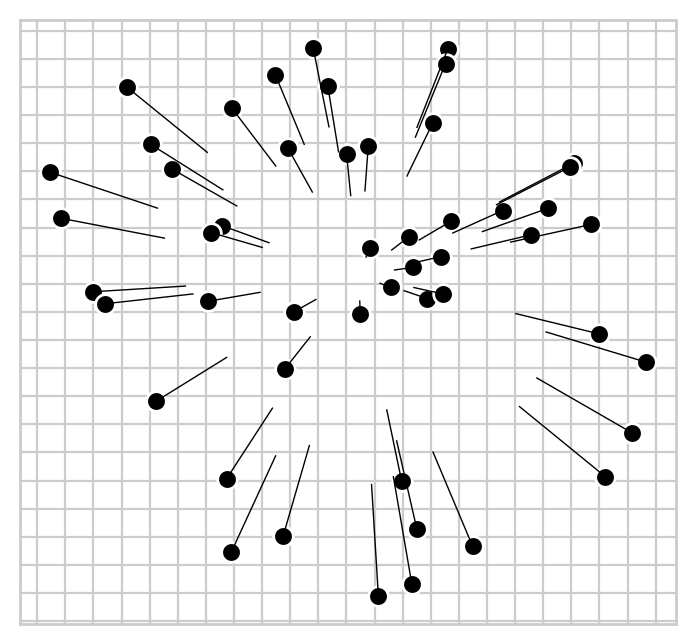# pymds¶

Metric multidimensional scaling in python.

## Installation¶

Use pip:

pip install pymds


## Background¶

Multidimensional scaling aims to embed samples as points in n-dimensional space, where the distances between points represent distances between samples in data.

In this example, edges of a triangle are specified by setting the distances between three vertices a, b and c. These data can be represented perfectly in 2-dimensions.

import pandas as pd
from pymds import DistanceMatrix

# Distances between the vertices of a right-angled triangle
dist = pd.DataFrame({
'a': [0.0, 1.0, 2.0],
'b': [1.0, 0.0, 3 ** 0.5],
'c': [2.0, 3 ** 0.5, 0.0]},
index=['a', 'b', 'c'])

# Make an instance of DistanceMatrix
dm = DistanceMatrix(dist)

# Embed vertices in two dimensions
projection = dm.optimize(n=2)


In data where distances between samples cannot be represented perfectly in the number of dimensions used, residual error will exist among the distances between samples in the space and the distances in the data.

Error in MDS is also known as stress.

## Usage¶

The following example demonstrates some simple pymds features.

from pymds import DistanceMatrix

from numpy.random import uniform, seed
from scipy.spatial.distance import pdist, squareform

import seaborn as sns
sns.set_style('whitegrid')

# 50 random 2D samples
seed(1234)
samples = uniform(low=-10, high=10, size=(50, 2))

# Measure pairwise distances between samples
dists = squareform(pdist(samples))

dists_shrunk = dists * 0.65

# Embed
original = DistanceMatrix(dists).optimize()
shrunk = DistanceMatrix(dists_shrunk).optimize()

shrunk.orient_to(original, inplace=True)

original.plot(c='black', edgecolor='white', s=50)
original.plot_lines_to(shrunk, linewidths=0.5, colors='black')